### Home > AC > Chapter 7 > Lesson 7.2.2 > Problem7-66

7-66.
1.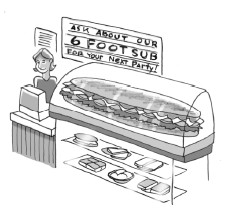Salami and More Deli sells a 6-foot sandwich for parties. It weighs 8 pounds. Assume the weight per foot is constant. 7-66 HW eTool (Desmos). Homework Help ✎

1. How much does a sandwich 0 feet long weigh?

2. Draw a graph showing the weight of the sandwich (vertical axis) compared to the length of the sandwich (horizontal axis). Label the axes with appropriate units.

3. Use your graph to estimate the weight of a 1-foot sandwich.

4. Write a proportion to find the length of a 12-pound sandwich.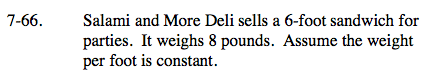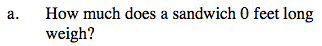0 pounds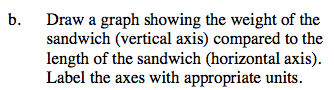Graph starting with the point where x = 0

Your graph should show a line with positive slope.
Units on the vertical axis should be in pounds.
Units on horizontal axis should be in feet.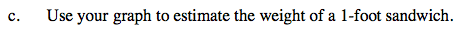What is the value of y when x = 1?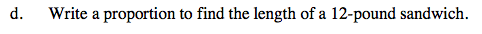Compare sandwich length and height.

$\frac{6}{8}=\frac{\it x}{12}$

9 feet